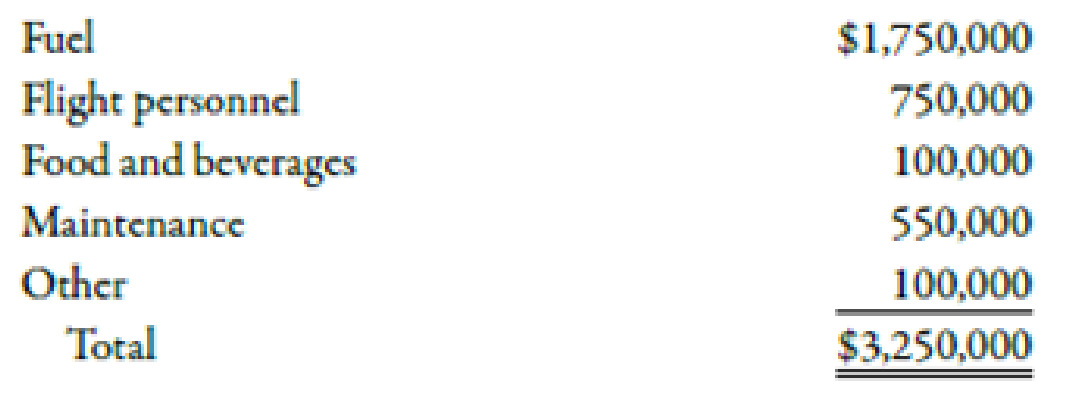Chapter 12, Problem 44P### Managerial Accounting: The Corners...

7th Edition
Maryanne M. Mowen + 2 others
ISBN: 9781337115773

#### Solutions

Chapter
Section### Managerial Accounting: The Corners...

7th Edition
Maryanne M. Mowen + 2 others
ISBN: 9781337115773
Textbook Problem
27 views

# Net Present Value, UncertaintyOndi Airlines is interested in acquiring a new aircraft to service a new route. The route will be from Tulsa to Denver. The aircraft will fly one round-trip daily except for scheduled maintenance days. There are 15 maintenance days scheduled each year. The seating capacity of the aircraft is 150. Flights are expected to be fully booked. The average revenue per passenger per flight (one-way) is $235. Annual operating costs of the aircraft follow:The aircraft will cost$120,000,000 and has an expected life of 20 years. The company requires a 12% return. Assume there are no income taxes.Required: 1. Calculate the NPV for the aircraft. Should the company buy it? 2. In discussing the proposal, the marketing manager for the airline believes that the assumption of 100% booking is unrealistic. He believes that the booking rate will be somewhere between 70 and 90%, with the most likely rate being 80%. Recalculate the NPV by using an 80% seating capacity. Should the aircraft be purchased? 3. Calculate the average seating rate that would be needed so that NPV will equal zero. Round the seating rate to the nearest percent. 4. CONCEPTUAL CONNECTION Suppose that the price per passenger could be increased by 10% without any effect on demand. What is the average seating rate now needed to achieve an NPV equal to zero? What would you now recommend? Round the seating rate to the nearest percent.

1.

To determine

Find out the NPV of the aircraft. Also, recommend that the company should buy it or not.

Explanation

Net Present Value:

The remaining balance of the present value of a project’s inflows and outflows is known as net present value (NPV). It is a discounting model of capital investment decision. A project with a positive NPV increases the wealth of a firm whereas a project with a negative NPV decreases the wealth of a firm.

Use the following formula to calculate NPV of the investment:

NPV=Presentvalueofcashinflow(P)Presentvalueofcashoutflow(I)

Substitute $160,032,7521 for P and$120,000,000 for I in the above formula.

NPV=$160,032,752$120,000,000=$40,032,752 Therefore, net present value of the investment is$40,032,752.

The company should buy the aircraft because NPV is positive.

Working Note:

1. Calculation of expected cash flow:

Calculation of days of operation:

Daysofoperation=TotaldaysMaintenancedays=365days15days=350days

Calculation of revenue per year:

Revenueperyear=Re

2.

To determine

Find out the NPV of the aircraft if the booking is 80% of seating capacity.

3.

To determine

Calculate the seating rate at which NPV will equal zero.

4.

To determine

Calculate the seating rate at which NPV will equal zero and the price per passenger is increased by 10%. Also state the recommendations.

### Still sussing out bartleby?

Check out a sample textbook solution.

See a sample solution

#### The Solution to Your Study Problems

Bartleby provides explanations to thousands of textbook problems written by our experts, many with advanced degrees!

Get Started

#### Find more solutions based on key concepts